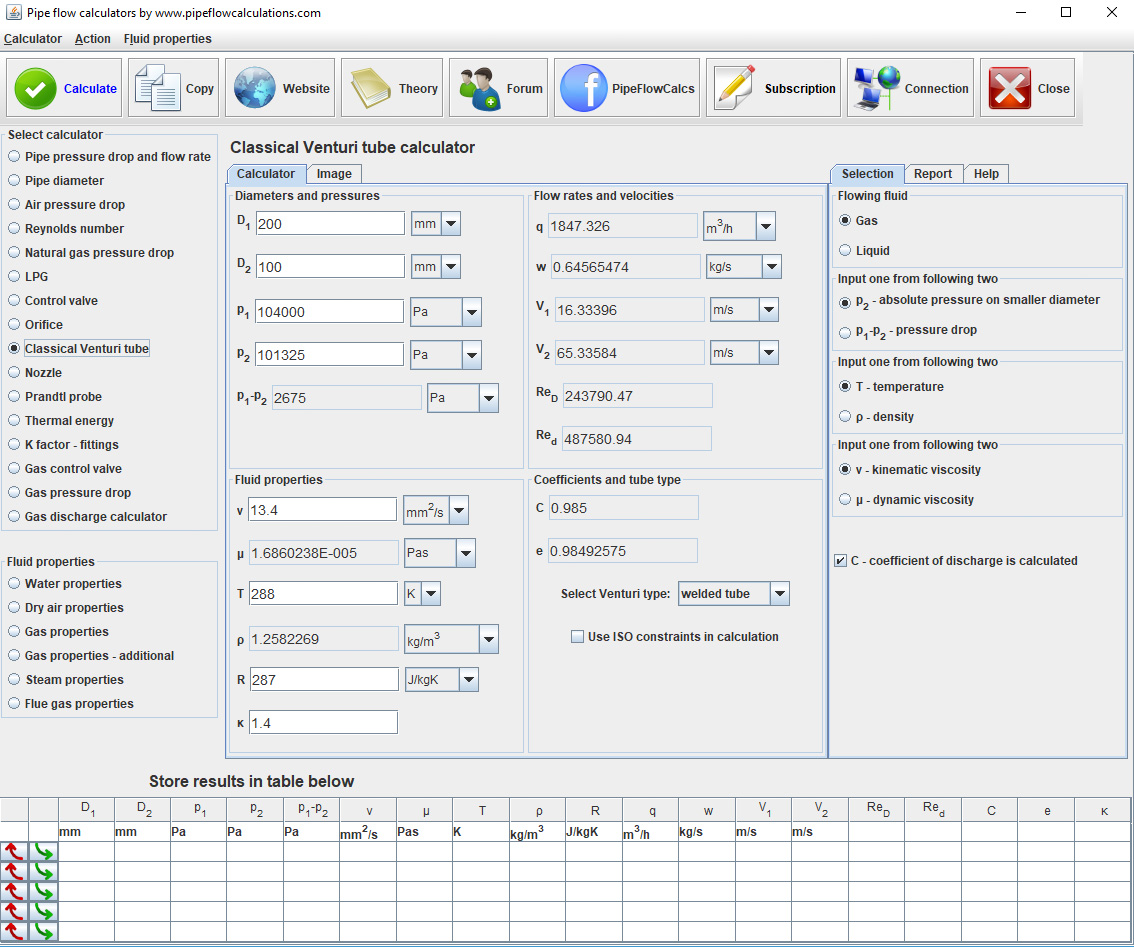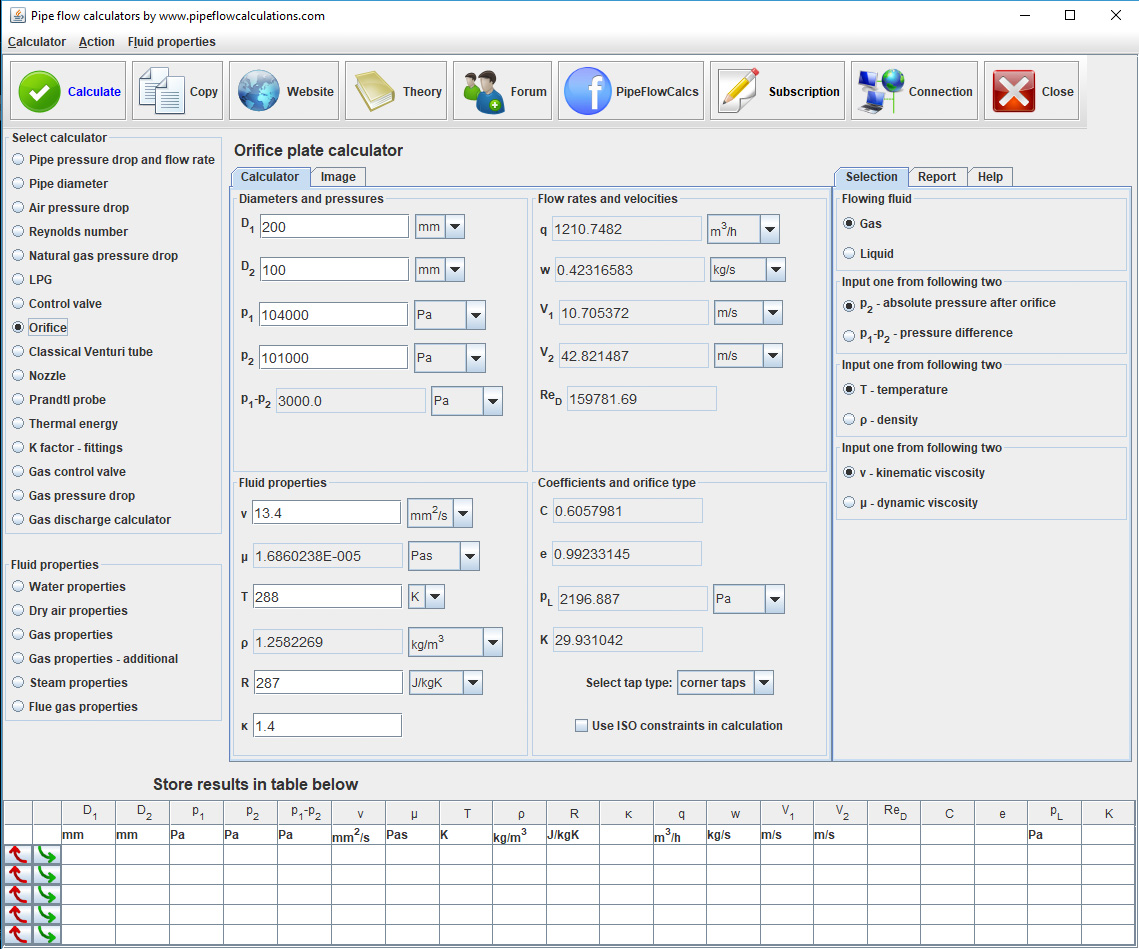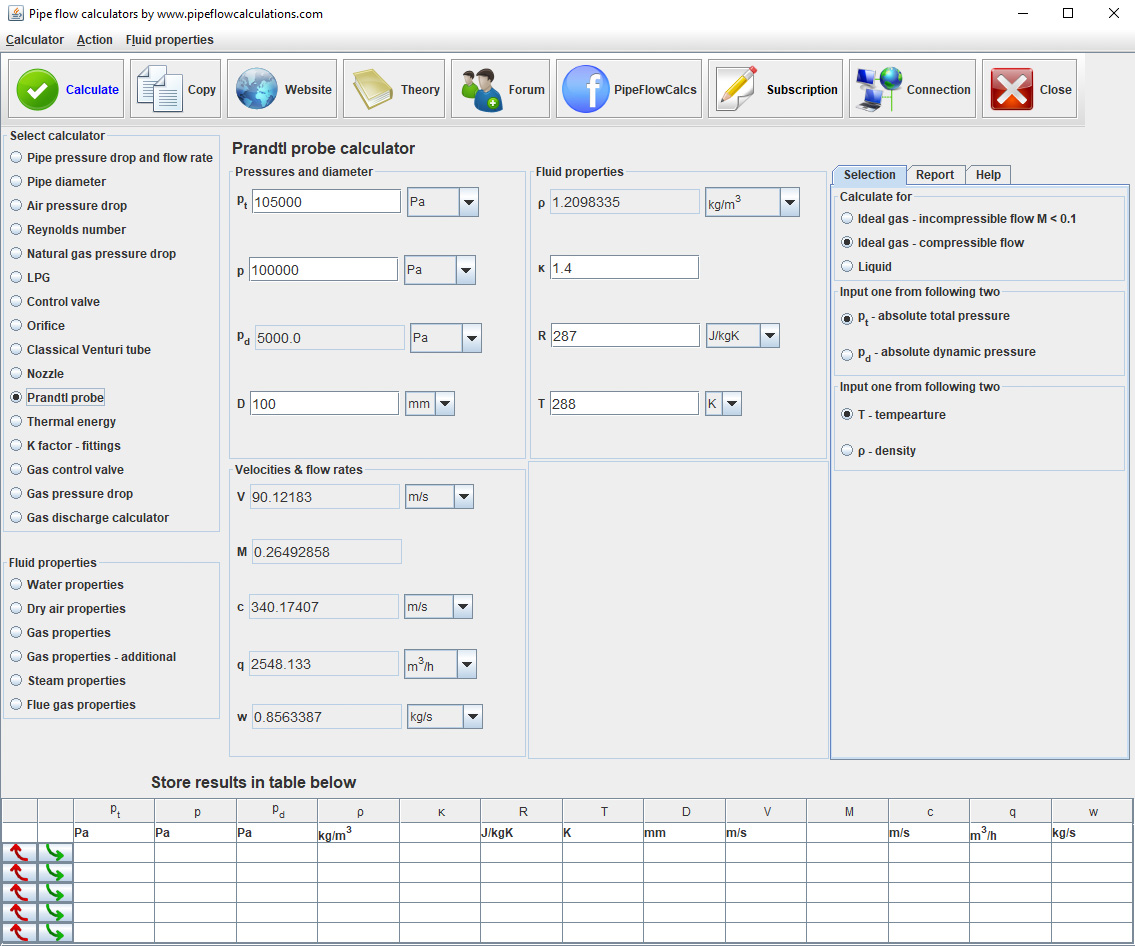# Nozzle and Venturi nozzle flow calculator

## flow rate measurement calculator

### online since 2011## Registration and subscription

Please login for full calculator service. Use the form on the top of the page.
Register an account if you don't have one.
Active subscription is required for full services.
Action
Calculation setup
Pressures
Flow rates and velocities
Nozzle dimensions and coefficients
Fluid properties

# Calculator description

Read more about calculator application and check if calculator is suitable for your problem.

Also, read about calculation procedures, what you have to know for the calculations and what limitations apply.

# Registration and subscription

Subscribed users have access to the full service which includes:

• One click switch from metric to imperial units
• Export results in word or excel format
• Preview, copy and paste results from a web page to your text editor
• Send results to you email

## Calculator start selection

Read all about available deployments. In any way of utilizing calculator, Internet connection is required, for authentication at least.

## Issues and answers

Having problems with calculators usage? Read more about known issues and problems and way to solve it.

#### Description

p1 - inlet pressure
Absolute pressure in front of the nozzle
p2 - throat pressure
Absolute pressure at the nozzle throat
Δp - pressure drop
Measured pressure difference
Δω - pressure loss
That can't be recovered after the nozzle
D1 - inlet diameter
Nozzle internal inlet diameter
D2 - throat diameter
Nozzle throat diameter
e - expansion factor
Coefficient used for compressible flow calculation
C - coefficient of discharge
Coefficient used for nozzle based on ISO 5167
ReD - inlet Reynolds
Reynolds number calculated in front of the nozzle
Red - throat Reynolds
Reynolds number calculated at the nozzle throat
K - resistance coefficient
That you can use in pipeline calculation, as nozzle is creating local pressure losses in the pipeline
q1 - volumetric flow rate
Fluid flow rate in terms of units of volume per unit of time on the inlet conditions
q - standard flow
Flow rate at standard conditions. Used only if the selected fluid is gas.
ṁ - mass flow rate
Fluid flow rate in terms of units of mass per unit of time
V1 - upstream velocity
Flow velocity at the nozzle inlet where flow diameter is D1
T1 - upstream temperature
Fluid temperature for gas density calculation based on the ideal gas state equation
ρ1 - upstream density
Fluid density at the nozzle inlet in terms of mass per unit of volume
R - gas constant
Gas constant in terms of energy per unit of mass and temperature, for gas density calculation using ideal gas state equation
κ - isentropic coefficient
Specific heat ratio
ν - kinematic viscosity
Result of fluid particles colliding to each other and moving at different velocities in terms of area per square unit of time
μ - dynamic viscosity
Result of fluid particles colliding to each other and moving at different velocities in terms of mass per square unit of distance and time

#### Calculation setup

Select nozzle type based on its shape
Nozzle
Classic shape
For less pressure drop
Venturi nozzle
Shaped like Venturi
Select value to input. You should enter selected one. The other one will be calculated
p2
pressure downstream from the nozzle
Δp
measured pressure difference in front and after the nozzle
Select value to input. You should enter selected one. The other one will be calculated
ν
kinematic viscosity
μ
dynamic viscosity
Select value to input. Available only if gas is selected. You should enter selected one. The other one will be calculated
T1
upstream temperature.
ρ1
upstream density.
Select fluid type
Gas
for compressible flow where ideal gas state equation is applicable
Liquid
for incompressible flow of liquids
Application of ISO 5167 constraints
ISO constraints
Deselect if you don't want to check if the nozzle and the flow conditions are within the limits of the ISO standard.

#### Related calculators## Venturi## Orifice